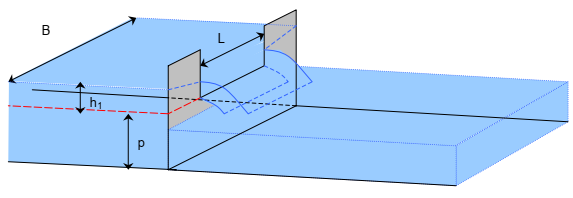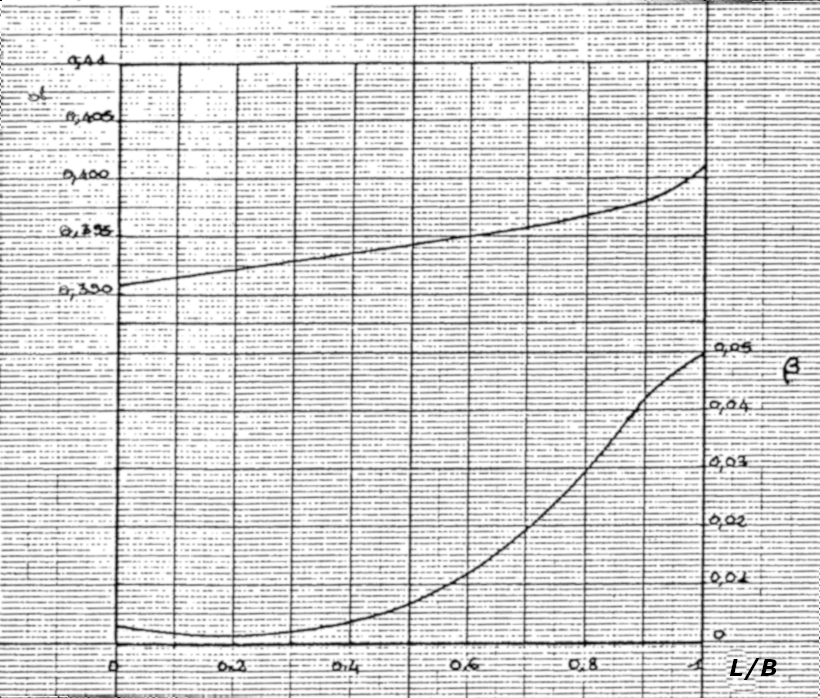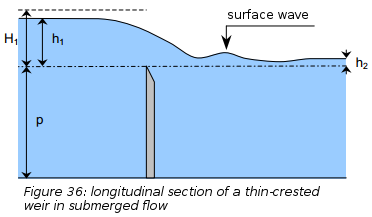# Kindsvater-Carter and Villemonte formula

The calculation module allows hydraulic calculations to be carried out for several structures in parallel.

## Kindsvater-Carter formula (1957)Perspective view of a rectangular weir with lateral contraction from CETMEF (2005)1

Kindsvater-Carter formula corresponds to the classic weir formula:

$Q = \mu L \sqrt{2g}h_1^{1.5}$

With:

• $$\mu$$ the discharge coefficient $$\mu = \alpha + \beta h_1/p$$
• $$L$$ the width of the weir
• $$h_1$$ the water level above the weir crest
• $$p$$ the sill or weir crest height

The coefficients $$\alpha$$ and $$\beta$$ depend on the ratio between the width of the weir ($$L$$) and the width of the basin ($$B$$). Their values are given in the abacuses below (Excerpt from Larinier, M., Porcher, J.-P., 1986. Programmes de calcul sur HP86 : hydraulique et passes à poissons):## Submerged flow: Villemonte formula (1947)Excerpt from CETMEF (2005)1

For a downstream water elevation higher than the elevation of the weir crest, the flow is submerged and a flooding coefficient is applied to the flow coefficient.

Villemonte proposes the following formula:

$K = \frac{Q_{submerged}}{Q_{free}} = \left [ 1- \left ( \frac{h2}{h1} \right)^n \right]^{0.385}$

With: - $$h_1$$ the upstream water level above the weir crest - $$h_2$$ the downstream water level above the weir crest - $$n$$ the exponent in free flow relationships (rectangular=1.5, triangular=2.5, parabolic=2)

1. CETMEF. Notice sur les déversoirs : synthèse des lois d’écoulement au droit des seuils et déversoirs. Compiègne: Centre d’Études Techniques Maritimes Et Fluviales, 2005. http://www.side.developpement-durable.gouv.fr/EXPLOITATION/DEFAULT/doc/IFD/IFD_REFDOC_0513410/notice-sur-les-deversoirs-synthese-des-lois-d-ecoulement-au-droit-des-seuils-et-deversoirs